# Tuning a DC motor speed controller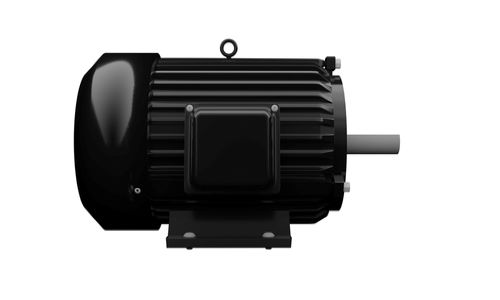This example will show how to model a DC motor's speed using different approaches in Collimator. The three approaches involve simulating diagrams from a differential equation, a transfer function, and a state variable model. Then, we will design and simulate a PID controller for the DC motor speed.

## DC variable speed motor overview

A DC motor combines mechanical and electrical subsystems. The electrical part is an armature coil with a resistance and inductance to drive a field causing motion. The mechanical part can be modeled as an inertial rotor with friction. The DC motor is conceptually modeled as in Figure 1 with the parameters given in Table 1.

Table 1 DC motor model parameters:

\begin{array} {|l|l|}\hline Symbol & Description & Value & Unit \\ \hline R & \text{Shunt Resistance} & 4 & \Omega \\ \hline L & \text{Coil inductance} & .25 & H \\ \hline K & \text{Motor Electromotive/torque constant} & .05 & N.m/A \\ \hline j & \text{Rotor intertia} & .02 & Kg.m2 \\ \hline b & \text{Friction constant}  & 0.1 & N.m.s \\ \hline  \end{array}

## DC variable speed motor model

The DC motor dynamics are given as the following system of differential equations:

$$L\frac{di}{dt} + Ri + K\frac{dϕ}{dt} = u$$

$$J\frac{d^2ϕ}{dt^2} +b \frac{dϕ}{dt} = Ki$$

Applying the Laplace transform for the above equations to get the domain equivalent as:

$$(Ls+R)I(s)+K\dotΦ(s) = U(s)$$

$$(Js+b) \dotΦ (s) = KI(s)$$

Then, both equations are combined by eliminating the current variable to get the open-loop transfer function as:

$$\frac{\dotΦ(s)}{U(s)} = \frac{K}{LJs^2+ (RJ +Lb)s + (Rb + K^2 )}$$

The transfer function can also be converted into an equivalent state space form as:

Equation 6

$$\frac{d}{dt}\begin{bmatrix}Φ\\\dotΦ\\i\end{bmatrix} = \begin{bmatrix}0 & 1 & 0\\0&-\frac{b}{J} &\frac{K}{J}\\0 & -\frac{K}{L} &-\frac{R}{L}\end{bmatrix}\begin{bmatrix}Φ\\\dotΦ\\i\end{bmatrix}+\begin{bmatrix}0\\0\\\frac{1}{L}\end{bmatrix}u$$

Equation 7

$$y=\begin{bmatrix}0 & 1 & 0\end{bmatrix}\begin{bmatrix}Φ\\\dotΦ\\i\end{bmatrix}$$

## Simulating Models

### Differential Equations Model

Substituting the parameter values in Table 1 within Equations $$L\frac{di}{dt} + Ri + K\frac{dϕ}{dt} = u$$ and, $$J\frac{d^2ϕ}{dt^2}+b\frac{dϕ}{dt} = Ki$$ , the DC motor speed model as a system of differential equations is described as:

$$0.25\frac{di}{dt} + 4i+ 0.05\frac{dϕ}{dt} = u$$

$$0.02\frac{d^2ϕ}{dt^2} + 0.1 \frac{dϕ}{dt} = 0.05i$$

The dataflow of the system of differential equations can be formulated as a simulation diagram in Collimator's model editor as shown in Figure 2.

The parameters of the models are defined in Figure 3.

After running the simulation, the step response is visualized as in Figure 4.

### Transfer Function Model

The obtained transfer function for the DC motor is defined as:

$$\frac{\dotΦ(s)}{U(s)} =\frac{0.05}{0.005s^2+ 0.105s + 0.4025}$$

The transfer function model is implemented as in Figure 5 with the transfer function block is configured as in Figure 6. The visualization of the step response of the transfer function model is shown in Figure 7.

### Simulating State Space

By substituting the DC motor model parameters with the state space model of Equations shown in Equation 6 and Equation 7, the following models are obtained:

From Equation 6:

$$\frac{d}{dt}\begin{bmatrix}Φ\\\dotΦ\\i\end{bmatrix} = \begin{bmatrix}0 & 1 & 0\\0& -5 & 2.5\\0 & -0.2 & -16\end{bmatrix}\begin{bmatrix}Φ\\\dotΦ\\i\end{bmatrix}+\begin{bmatrix}0\\0\\4\end{bmatrix}u$$

From Equation 7:

$$y=\begin{bmatrix}0 & 1 & 0\end{bmatrix}\begin{bmatrix}Φ\\\dotΦ\\i\end{bmatrix}$$

The state space model is implemented in Figure 8 and the state space block is configured in Figure 9. The visualization of the step response of the state space model is shown in Figure 10.

## DC motor speed controller design

The ideal parallel form the PID controller is defined as:

$$u(t)= K_pe(t)+K_i ∫_0^te(τ)dτ+K_d\frac{d}{dt}e(t)$$

The PID controller simulation model is implemented through Collimator's model editor as shown in Figure 11.

First, we try an initial PID tuning as $$K_p=K_i=K_d=1$$. The step response of the closed-loop DC motor speed with the initial PID design is illustrated in Figure 12.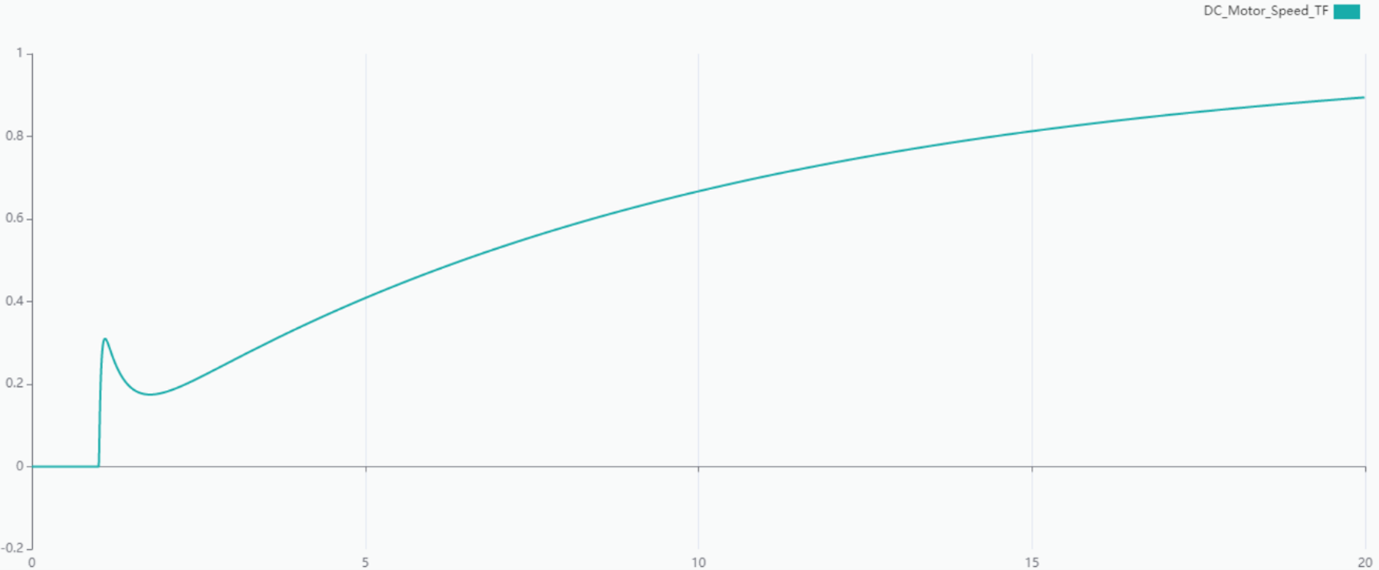Figure 12 Step response for PID with $$K_p=K_i=K_d=1$$

The initial PID tuning yields a very slow step response. Therefore, we increase the proportional gain to $$K_p=80$$ Then, the step response is plotted in Figure 13. The new PID controller has much faster response. However, there is a significant steady-state error about 9%.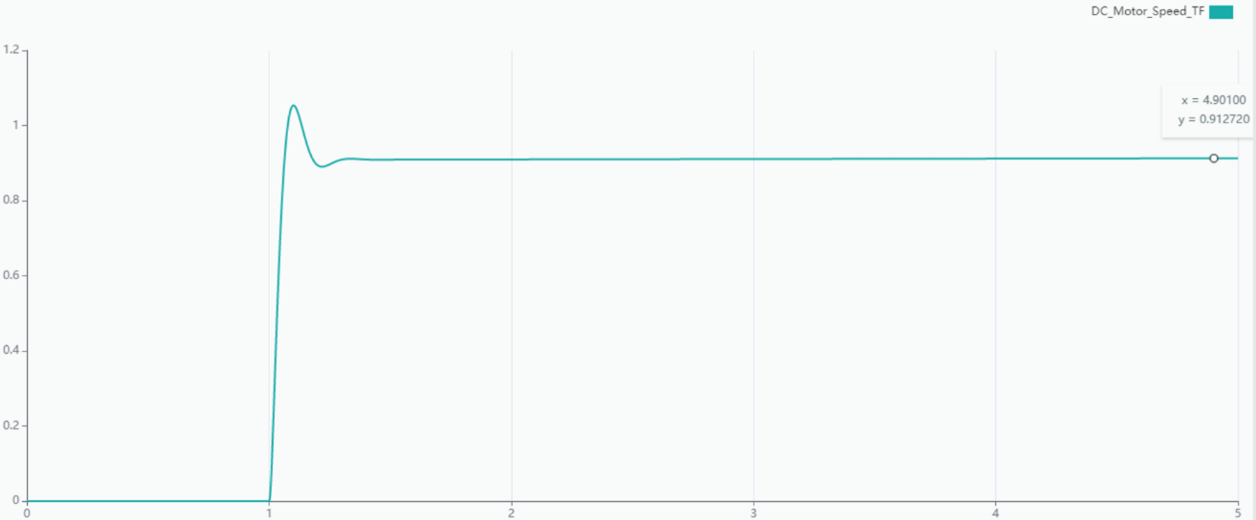Figure 13 Step response for PID with $$K_p=80,K_i=K_d=1$$

To eliminate the steady-state error, we increase the integral gain to $$K_i= 50.$$ This yields the step response in Figure 14. Now, the steady-state error is eliminated.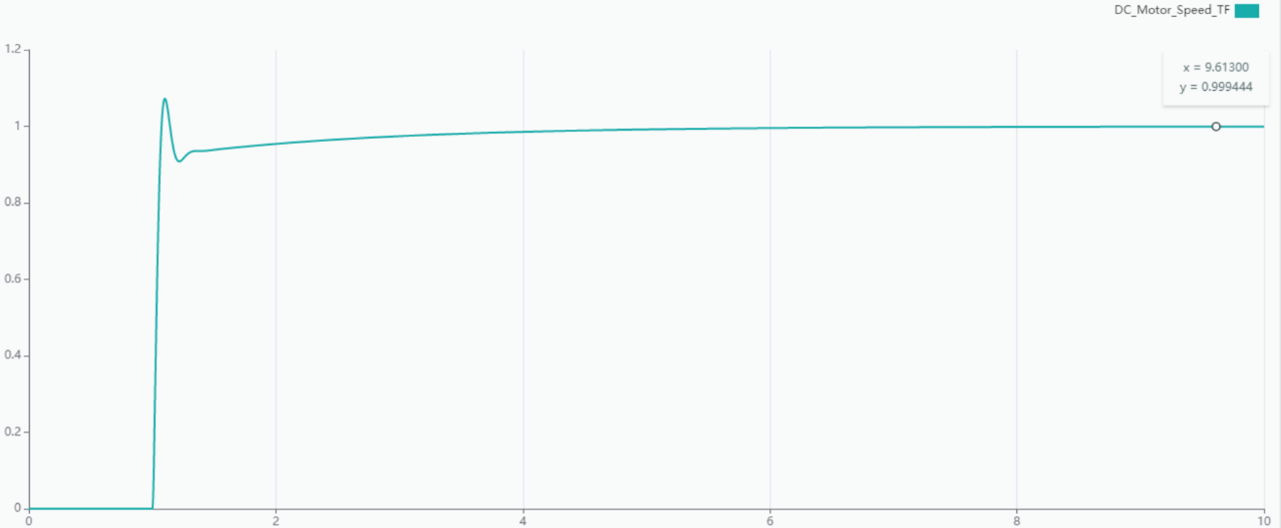Figure 14 Step response for PID with $$K_p=80,K_i=50,K_d=1$$

## DC motor speed control results

To accelerate the settling time, increase the $$K_d = 6.$$ The final step response is plotted in Figure 15.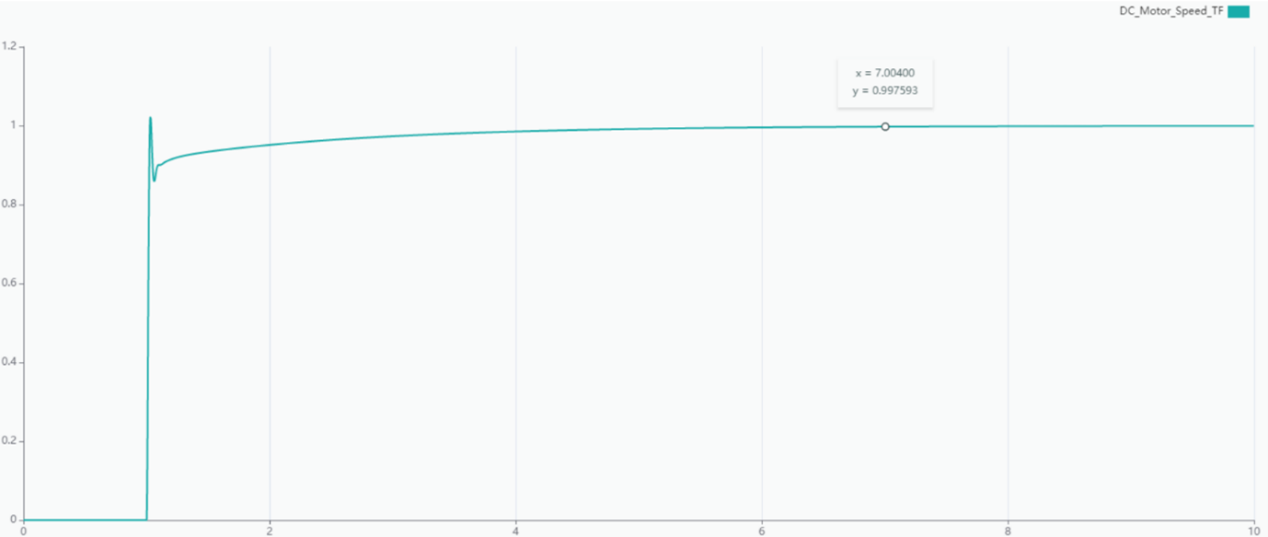Figure 15 Step response for PID with $$K_p=80,K_i=50,K_d=6$$